# What Is the Remainder?

Division won’t always give you a neat and clean answer – sometimes, you’ll be left with a remainder.Author
Taylor HartleyExpert Reviewer

Last updated: August 24, 2023# What Is the Remainder?

Division won’t always give you a neat and clean answer – sometimes, you’ll be left with a remainder.Author
Taylor HartleyExpert Reviewer

Published: August 24, 2023# What Is the Remainder?

Division won’t always give you a neat and clean answer – sometimes, you’ll be left with a remainder.Author
Taylor HartleyExpert Reviewer

Published: August 24, 2023Key takeaways

• Think of the remainder as a leftover – It’s like making 8 servings but only having 7 people over – you’ll have 1 serving remaining, right? The same goes for division.
• A remainder should always be smaller than a divisor – Remainders will always be less than the divisor (a term we will define later). If your remainder is greater, that means you need to keep dividing.
• Remainders can be expressed as fractions or decimals – Remainders are commonly expressed as a number following a capital R, but they can also be written in fraction or decimal form, too. Any fraction or decimal added to a whole number is considered a remainder.

Has your guardian ever made dinner for the whole family thinking they’d cooked just the right amount, only to discover they’ve got an awkward amount of food left over? Well, in math, you’ll occasionally encounter that exact same problem. Don’t worry, though. Leftovers often mean you’ve got lunch for the next day – and remainders usually mean you’ve done something right!

Today, we’ll take an in-depth look at remainders: what they are, when they happen, and what to do once you find one. At first, you might feel like you’ve made a mistake when you’re left with a remainder, but rest assured, you (probably) haven’t messed up. Let’s dive in!

## Important terms to know for divisionBefore we get going, there are a few key terms you’ll need to know to understand division. It’s tough talking about division if you don’t know these terms, so make sure you review them prior to continuing through this guide. You’ll need to know them as we talk about and practice finding remainders.

• Divisor – The number you are dividing something else by (so, if the problem reads 145 ÷ 5, the divisor is 5
• Dividend – The value being divided (in 145 ÷ 5, the divisor is 145)
• Quotient – The value you get after you divide the dividend by the divisor (145 ÷ 5 = 29)
• Remainder – The amount left over when one number does not divide exactly into another number. The remainder will always be less than the divisor (there is no remainder in 145 ÷ 5, but we will explore this more below)

## So, what is the remainder in math?Like the word suggests, a remainder is something left over, or “remaining” after you’ve completed a division problem. Once you’ve gone through all the steps of dividing, you might still have an extra value remaining. This always happens when one number is not completely – or evenly – divisible by another.

Don’t worry: you haven’t messed up! You’ve just reached the end of your calculation and it’s time to put your pencil down. That’s because remainders are part of the answer. Remember, the remainder should always be less than the divisor!

## Remainder formulaLook at the important terms to know. These terms are all key pieces of the division puzzle. You can determine the value of each of these pieces by using the others.

Take a look at this equation, which can help you quickly solve some of your problems if you have most of the pieces but need to find others:

Dividend = (Divisor x Quotient ) + Remainder

Use this basic formula to calculate the divisor, quotient, or remainder, depending on which pieces you have and which you still need to find.

You can also rewrite this equation to find the remainder. Just switch a few things around:

Remainder = Dividend — (Divisor x Quotient)

## How to find the remainder in 3 easy stepsUsing the equation above, we can calculate the value of our remainder in three easy steps.

### 1. Identify the different parts of the equationFirst, it’s important that we understand each of the values in our problem, and which terms correspond to each of those values. That means you’ll need to identify what number represents the divisor, the dividend, the quotient, and the remainder – and which values might be missing.

### 2. Check if the dividend is a multiple of the divisorNow, we need to take a look at our dividend (the number that’s being divided), and see if there’s a specific value (our quotient) we can multiply by the divisor to get the dividend.

If there is no exact number to multiply by, we simply multiply to find the next smallest multiple.

A multiple is a number that can be divided by another number without a remainder.

For example:

Consider 70 ÷ 4. 70 is not completely divisible by 4, but 4 x 17 = 68. 68 is the largest possible multiple of 4 not higher than 70, so 17 (the number we multiplied by) will serve as our quotient.

### 3. Subtract your largest multiple from the dividendNow that we’ve found the largest multiple, we can simply subtract it from our dividend. 70 – 68 = 2, so 2 will represent the remainder in our equation of 70 ÷ 4.

So, 70 ÷ 4 = 17 with a remainder of 2.

This can also be written as 17 R 2.

## The remainder in long divisionRemainders exist in long division, too. If you haven’t already reviewed our article on long division, make sure to check it out to get a more in-depth review prior to continuing.

The same basic steps apply.

1. Find the largest multiple of the divisor that fits into the dividend.
2. Subtract the corresponding digits of the dividend by that multiple.
3. Drag the next digit of the dividend down
4. Repeat, repeat, repeat.

The values multiplied by the divisor to calculate those multiples are written as digits that will eventually give you your quotient. When you have divided your divisor into the last digit of your dividend, there may still be a value left over that can no longer be divided.

And guess what? That is your remainder.

## Let’s practice together!### 1. A teacher decides to hand out stickers to her students. There are 134 stickers in total, and 20 students. If each student gets the same amount of stickers, how many stickers does each student get, and how many are leftover?1. Here, we can tell that the divisor will be the number of students (20), and the dividend will be the amount of stickers (134).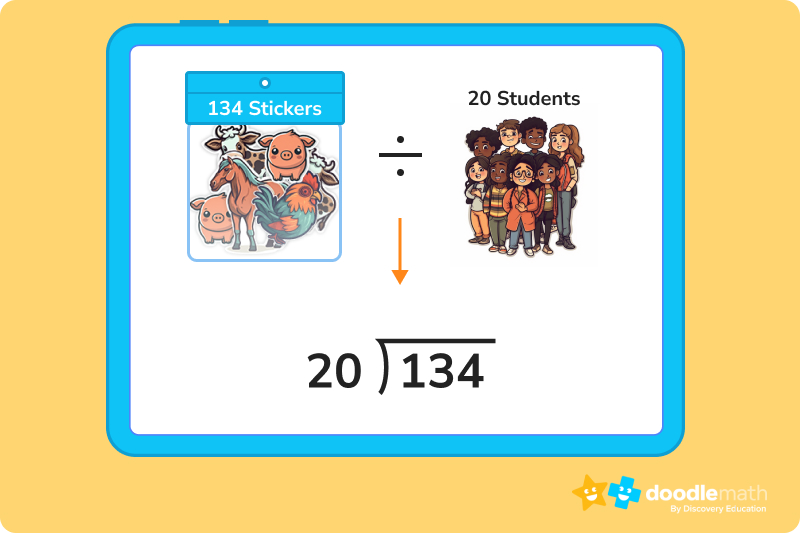2. We look to see how many times 20 goes into our first digit 1. It cannot divide into 1, so we look to see if it can be divided into our first two digits, 13. Again, it cannot be divided into this value, so we look to see if it can be divided into our first 3 digits, 134, which it can.

3. 20 fits into 134 six times. 20 multiplied by 6 equals 120, and 134 – 120 = 14.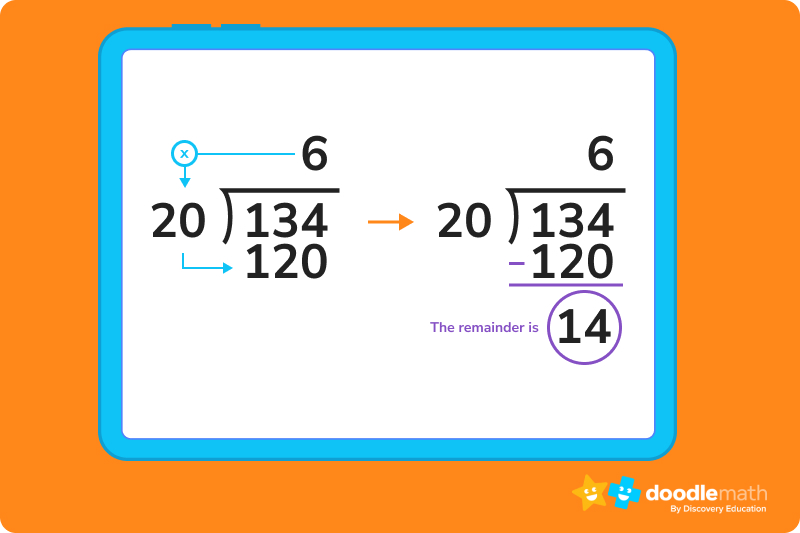So, we know that each student receives 6 stickers, and there are fourteen stickers left over.### 2. Calculate the value of the remainder with a dividend of 147, a divisor of 13, and a quotient of 11.1. Here, we can use the equation from earlier to easily calculate the remainder.

2. Just remember that to find the remainder, you just need to multiply the divisor and the quotient, and subtract that product from the dividend.

3. 11 x 13 = 143

4. 147 – 143 = 4

5. The remainder is 4.

### 3. What is the remainder when 245 is divided by 12?1. First, let’s define both the dividend and divisor. We’re dividing 245 by something else, so 245 is our dividend, and 12 is our divisor.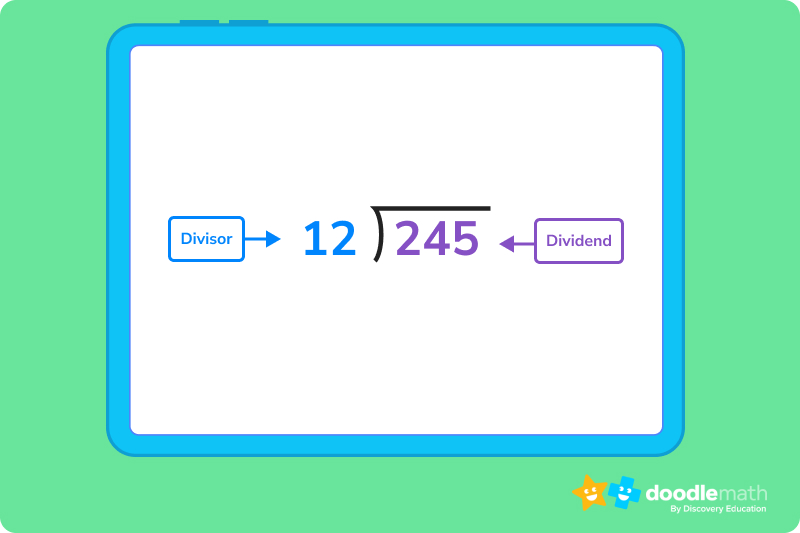2. Now, let’s compare the 12 with the first digit of 245. We know 2 does not go into 2, so we see if it goes into the first 2 digits of the dividend, 24. 2*12=24, so we put a 2 above the tens place in our quotient, multiply it out, subtract, then bring down the next digit.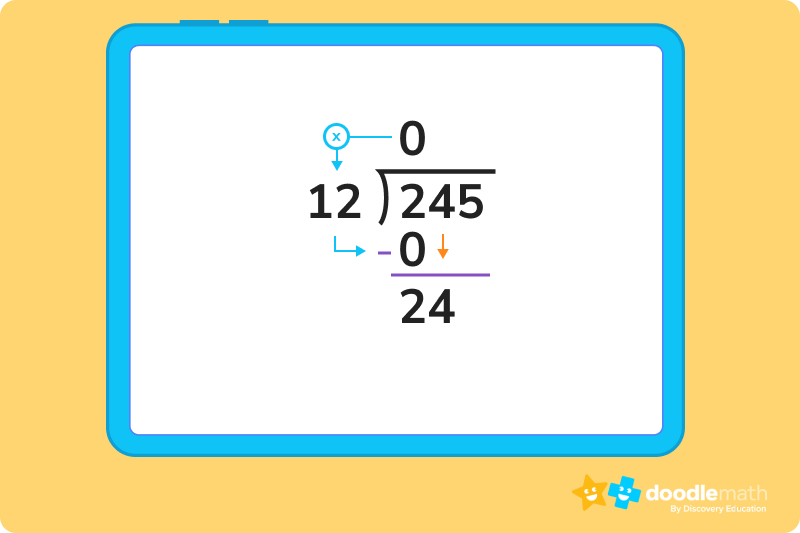3. 2 – 0 = 2, and we can then drag the 4 down next to this 2 to make 24. 24 is exactly divisible by 12, so we can write a 2 in the quotient.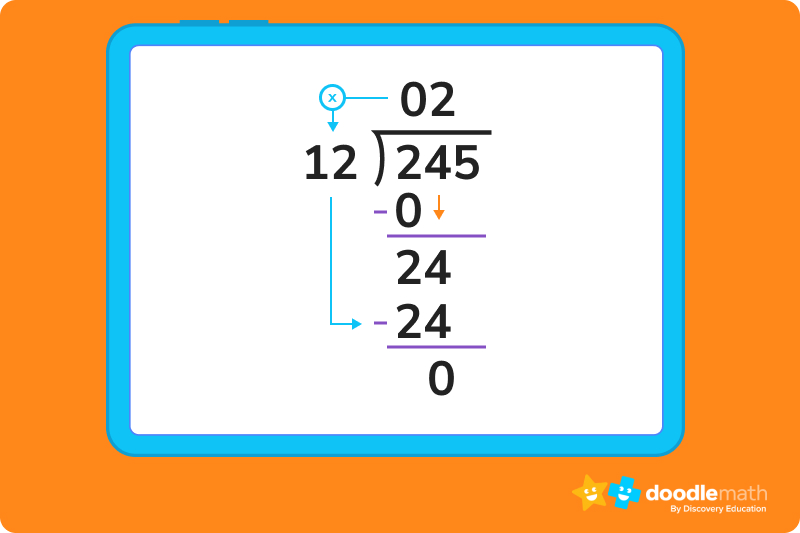4. 24 – 24 = 0, and now we can drag the 5 down next to this zero. 12 is larger than 5, so we place another 0 in our quotient.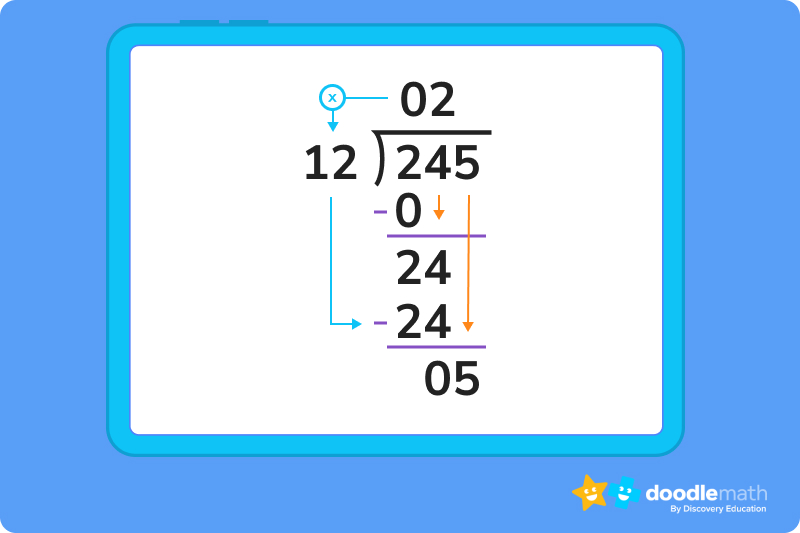5. We multiply the 0 by 12, and subtract that answer from the 5. 0-5=5, so we are left with a quotient of 20 with a remainder of 5, or 20 R 5.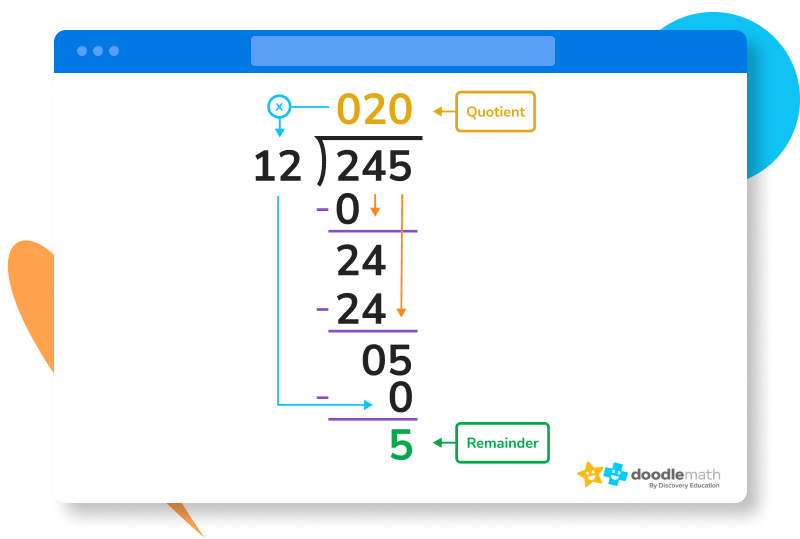Ready to give it a go?

Now that we’ve run through a few problems together, it’s time for you to get some practice in by yourself. Feel free to look back at the explanations, equations, and definitions above to help you along the way.

Try your best not to get discouraged! Finding the remainder isn’t always easy, but it’s an important piece of the long division puzzle that you’ll need to master. So keep trying!

## Practice problems

The remainder is 0.

Each friend gets \$12, and there is \$3 left over.

The remainder is 5.

The remainder is 9.

## Parent Guide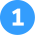The remainder is 0.

How did we get here?

1. We’ll start by comparing the divisor to the first digit of the dividend. 3 fits into 5 once, so we’ll place a one in the quotient and multiply it by 3.
2. We’ll subtract that product from 5. 5 – 3 = 2. Now we can drag the 4 down to create 24.
3. 3 fits into 24 eight times, so we write an 8 next to our one in the quotient, and multiply that 8 by 3.
4. 8 x 3 = 24, and 24 – 24 = 0. Since there is nothing left to drag down, we’re finished. 54 is perfectly divisible by 3, so the remainder in this problem is 0.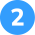Each friend gets \$12, and there is \$3 left over.

How did we get here?

1. First, we establish that we’re dividing 63 by 5.
2. 5 fits into 6 once, so a 1 goes in our quotient and is multiplied by our 5.
3. We then subtract 5 from 6, and get a 1. We can then drag our 3 down to give us 13.
4. 5 fits into 13 twice, so we write a 2 in the quotient, multiply it by 5, and subtract.
5. 13 – 10 = 3. Since there are no more digits to drag down, our division is finished. Each girl gets \$12, and there is \$3 to spare.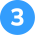The remainder is 5.

How did we get here?

1. 7 fits into 8 only once, so we can write a 1 in the quotient and multiply it by our 7. We then subtract this product from 8.
2. 8 – 7 = 1. Now we need to drag our 9 down next to the 1, giving us 19.
3. 7 fits into 19 twice, so we write a 2 in the quotient and multiply 2 by 7.
4. 2 x 7 = 14, and we subtract 14 from 19 giving us 5. There is nothing left to drag down, so the division is finished. We are left with a quotient of 12 and a remainder of 5.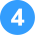The remainder is 14.

How did we get here?

1. For this equation, we can use the same equation from earlier in this article to calculate the remainder.
2. All we need to do is multiply the divisor (23) by the quotient (24), and subtract that product from the dividend (566).
3. 23 x 24 = 552.
4. 566 – 552 = 14, so our remainder is 14.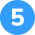The remainder is 9.

How did we get here?

1. The first digit of the dividend (1), is less than our divisor, so we write a 0 as our first digit in the dividend, multiply it by 10, and subtract that product from 1.
2. 1 – 0 = 1. We now drag down the 1 in the tens place, making an 11.
3. 10 fits into 11 only once, so we can write a 1 next to our 0 in the quotient.
4. 10 x 1 = 10, and 11 – 10 = 1. Now we drag down the 9 in the tens place of the dividend to make 19.
5. Tens also fits into 19 only once, so we write another 1 in the quotient, multiply it by 10, and subtract.
6. 19 – 10 = 9. There is nothing left to drag down, so our division is finished.
7. 119 ÷ 10 gives us a quotient of 11 and a remainder of 9.

## FAQs about math strategies for kidsWe understand that diving into new information can sometimes be overwhelming, and questions often arise. That’s why we’ve meticulously crafted these FAQs, based on real questions from students and parents. We’ve got you covered!

You might not always get an exact amount when performing short or long division. It’s important to understand how leftover values are created, and how you should think of them in relation to both the divisor and the dividend. Remainders are an essential part of understanding the majority of division problems you’ll encounter, as the majority of the time we divide the answer isn’t perfect. Remainders exist to provide more accurate quotients.

The remainder will always be less than the divisor. If your remainder is greater than your divisor, that means there is still more division you can perform, and your quotient is incomplete. If the remainder is greater than the divisor, that means the divisor can still fit into the dividend another time. Continue to divide until the divisor is greater than the remainder to get the most accurate answer.

The remainder will only ever be 0 when the dividend is perfectly divisible by the divisor. In other words, if the dividend is a multiple of the divisor, there won’t be any leftover values after dividing. In these situations, the remainder will be 0.

If you’re trying to calculate the remainder without performing standard long division, you can use the remainder theorem to find it instead. However, this relies on you knowing the values of the dividend, divisor, and quotient beforehand. The equation is the following:

Remainder = Dividend — (Divisor x Quotient)## Related Posts

Title 1

Title 2

Title 3

Lesson credits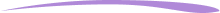Taylor Hartley

Taylor Hartley is an author and an English teacher based in Charlotte, North Carolina. When she's not writing, you can find her on the rowing machine or lost in a good novel.

Jill Padfield has 7 years of experience teaching high school mathematics, ranging from Alegra 1 to AP Calculas. She is currently working as a Business Analyst, working to improve services for Veterans while earning a masters degree in business administration.

Taylor Hartley

Taylor Hartley is an author and an English teacher based in Charlotte, North Carolina. When she's not writing, you can find her on the rowing machine or lost in a good novel.

Jill Padfield has 7 years of experience teaching high school mathematics, ranging from Alegra 1 to AP Calculas. She is currently working as a Business Analyst, working to improve services for Veterans while earning a masters degree in business administration.# Are you a parent, teacher or student?

Are you a parent or teacher?

## Hi there!

Book a chat with our team